Also: Manifolds of equilibria
Next: Twisted homoclinic bifurcation

# Manifolds of periodic orbits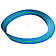The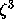model is the three-dimensional vector field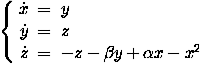with parameters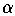and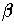. this system has two equilibria, one at the origin and one at B = (, 0, 0).

We take= 3.2 and= 2. For these parameter values, the point B is unstable due to a Hopf bifurcation at== 2. The attracting periodic orbit that appears in this Hopf bifurcation becomes of saddle type due to a period-doubling bifurcation. This means that one of its Floquet multipliers moved through -1 and the unstable manifold is, therefore, non-orientable. In fact, also the stable manifold of this periodic orbit is topologically a Möbius strip. The animations below show the stable and unstable manifolds.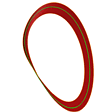The animated gif shows the unstable manifold rotating about the z-axis, centered at B (2.8MB).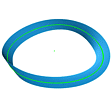The animated gif shows how the stable manifold grows, while it rotates about the y-axis, centered at B (766KB).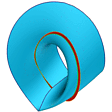Both manifolds rotating about the z-axis, centered at B (2.8MB).

The two-dimensional unstable manifold of the equilibrium (, 0, 0) accumulates on the attracting period-doubled periodic orbit. An impression of the structure of this manifold can be obtained by considering a Poincaré section and rotating this section over 360 degrees.

 Accumulation Two stills showing the period-doubled attractor and the unstable manifold of B accumulating on it. Heteroclinic intersection The unstable manifold of B forms a generic heteroclinic intersection with the non-orientable stable manifold of the periodic orbit.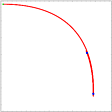The animated gif shows the unstable manifold of B = (, 0, 0) in a rotating Poincaré section. The attractor appears as a pair of period-two points in the section. Note how the Poincaré sections "glue" one point of the attractor to the other after one rotation (215KB).

Next: Twisted homoclinic bifurcation
Also: Manifolds of equilibria